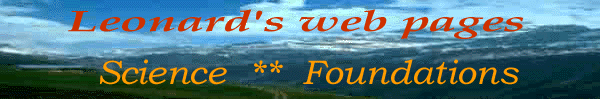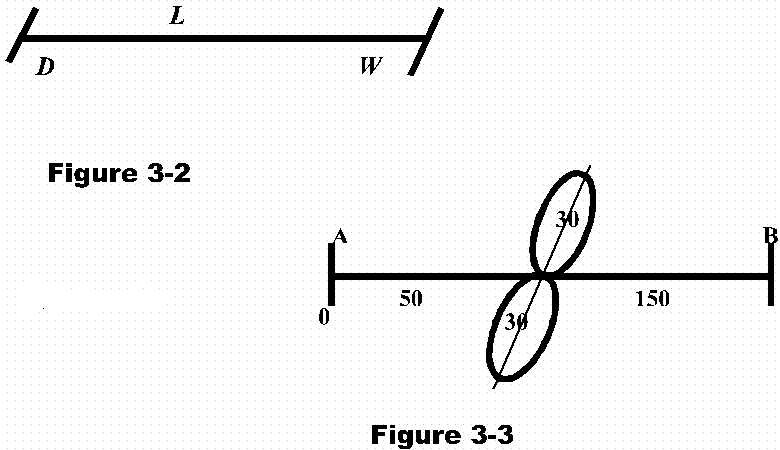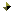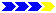# CHAPTER  3INDEX TO OTHER PAGES

1. Indescribable is what space is, for to say "empty," what "is emptiness" other than nothingness?  Or what is nothingness more fundamental than the absence of material being?

2. We cannot give geometrical forms or dimensions to space, nor to time, since space, as such and time also in its context, are considered a void.  And "a void" as such cannot be curved for how shall one bend emptiness?

3. Nor are there dimensions to the fourth or fifth as some allow their imagination to be.  An object resting on a sheet that is stretched between two points may curve the sheet, but there is no third dimension except such as already exist.  Moreover, what shall we call the poles by which the sheet is held?

4. Placing an object in a bowl of water displaces the water to its three dimensional form.  But as to "matter" - the nature of dust that is - when it is in space, does not displace space, but fills the space, or utilizes the same.  And thus there is a distinct difference that at fundamental levels cannot be ignored.

5. Nature now is three-dimensional.  There is matter, motion, and the coordinate factor.  Or in the spatial measures, longitude, latitude, and the vector range.  Or length, width, and depth.  These dimensions are, and bring indeed values, and no other dimension exist within this nature, nor is there any other dimension so relevant.

6.   But if we come to speak of what is beyond this nature, then we do not speak of another dimension, but rather of another "nature".  For as I have seen there are other natures in addition to our physical nature that co-exist within the same something we call space.  But O what a fantasy man in his ignorance may make of these words that I speak.1. The three dimensions of mass, when graphically stated, would be as illustrated by figure 3-1.  But I took up this subject to show something much more fundamental.  For ask yourself how this nature came to be in its three-dimensional form, and what is so fundamental thereto?

2. Where does it begin and where may it leave off?  How for example is its depths fashioned, and at what measure?  For we do have measures, we have three measures, three dimensions, where thus do they begin and end?

3.   Now that we have the question, we can say "well they all begin with zero, and from there into infinity."  But what makes "zero" a beginning or infinity an end?  Did we not take the zero and divided the same to fractions that go into infinity as well?  And thus "where" is the point of a beginning?

4. Where is the entry into a perfect circle?  .  After taking a good look at a ring, or any full circle, we have little choice but to confess that there is neither a beginning nor end.  And yet we did have a beginning, and so did the entire universe and this nature of dust.

5. Are my questions beyond conception, too far-reaching?  Perhaps so, for our knowledge is much too limited to step beyond such borders.  Nevertheless, allow me to probe where men not usually probe.  (Figure3-2)1.   Let us have width and length, with depth added thereto.  For the line, in any length has a width no matter how minute that width may be, or how thick that width might be.  For while the narrowest line still has width, the thinnest line has depth.

2. Therefore, to strike only a length we will strike all three dimensions simultaneously.  It thus is impossible to have any one without the other.

3. And then again how can we say to have three when it is one or one when it is three?  The absolute necessity of "one being for the other" is enhanced here.  And the fact that three is the division of "one" may also be clarified to some point.

4. But this is not the reason I took to speak on this subject here, but rather to begin to convey the fundamentals of nature to its three dimensions.  And so mark that a three-point principle is the difference of one rather than of three, nor shall it be one added to two, but two added to one.

5. And while this may seem somewhat superficial for the moment, I will remark that we have touched upon one of the most beautiful things in nature and also one most complex to understand.  And since I intend to speak once again on this under a heading of "Pololoid" it is here to a different approach.

6.   So once again let us strike a line (Figure 3-3) and let us enlarge the width and depth of that line in some marking upon it.  What is it that we should draw?  Yes indeed, what should we draw?  We would first draw a line at right angle to signify width, then another at some angle to signify depth.

7.  But in nature this is not so, since width and depth are one and the same with length as were it a circle in which infinity is absolute, since there is no beginning nor end to be found.

8. Nor can we draw a circle to signify the three dimensions since there is "linear motion" as well as circular, and the linear aspect is as real as the other.

9.   We therefore must show a linear which in that respect shows a beginning and an end, and upon which the expanse of nature may be placed.

10. For here the secret to find a beginning in infinity is to take the circle and twist it one half turn to become a figure eight, in which case there is both a point of intersecting, and a linear born forth of the fundamental circular formation.

11. The linear line then is placed upon this point of intersecting, and for its expanse can be divided to a measure of 200, 100 on each side of the centering.  Or to put it another way, we place the three dimensions upon the middle of the line.

12.To thus divide one in three is also to find a point of entrance into the circle, or a beginning in what by figure of speech may be said, "eternity", or infinity.

13.   It is at the point of intersecting then that we find our nature, the nature of dust.  The point where molecular motion slows down, where meats no longer spoil, and where to the left, zero temperature may be found, and where - to the right - temperature increases.

14. Accordingly we find our place in the nature of our bodies which ranges some 60 numbers, 30 to each side of the center.  (see Pololoid >page 17)

15. Thus we have the dimension of nature in its single being and the entrance into the circle, and the expanse of these dimensions, crude but accurate. Accordingly here is the first of several priceless gems.

16. But how am I to expand upon it when man does not even acknowledge receipt of my letters let alone remark upon them?  So thus man has shortchanged himself, and I will close my mouth as well as button my shirt for him.Next page